Need Help?

Subscribe to Circuit

###### \${selected_topic_name}
• Notes
• Comments & Questions

$\begin{array}{l}{\text { The switch in the circuit shown in Fig. has been in positiona for a long time. At } t=0 \text { , the switch moves from }} \\ {\text { position a to position } b \text { . The switch is a make-before-break type; that is, connection at position } b \text { is established }} \\ {\text { before the connection at position a is broken, }} \\ {\text { so there is no intersion at position a is broken, }} \\ {\text { a) Find the spression for } i(t) \text { for } t \geq 0 .} \\ {\text { b) What is the initial voltagacross the inductor }} \\ {\text { just after the switch has been moved to position b? }}\end{array}$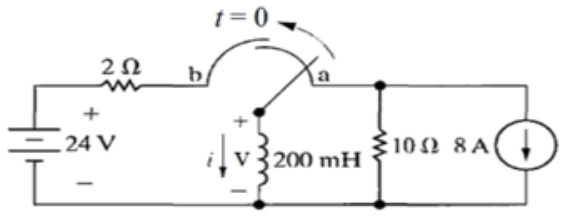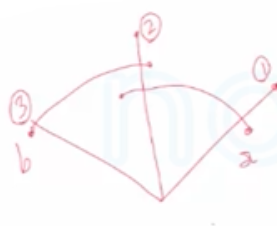خطوات الحل

(1) نحدد $I_0$ قبل تغير المفتاح

(2) نحسب $\tau$

(3) نعوض في علاقات $I, V$

$t<0$
$\text { switch at position } a$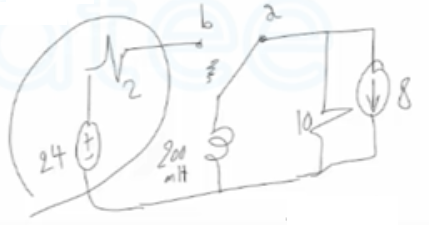$D C \quad \frac{d i}{d t}=0 \quad V_{L}=0 \quad {\Rightarrow S \cdot C}$

$I_{0}=-8 A$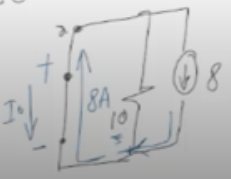$t<0$
$\text { switch at position b }$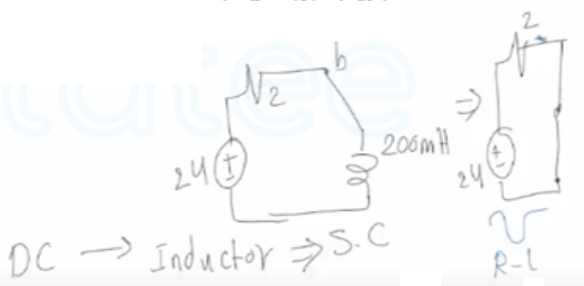$R=2 \Omega$, $V_{s}=24 \mathrm{V}$, $\tau=\frac{L}{R}=\frac{200 * 10^{-3}}{2}=0.1 \mathrm{sec}$

$i_{l}(t)=\frac{V_{s}}{R}+\left(I_{0}-\frac{V_{s}}{R}\right) e^{\frac{-t}{\tau}}$

$i_l(t)=\frac{24}{2}+[-8-\frac{24}{2}]e^{\frac{-t}{0.1}}=12=20e^{-10t}$

$t \geq 0$

$V_{l}(t)=L \frac{di(t)}{d t}$

$V_{L}(t)=200*10^{-3} *[-20*-10e^{-10t}]$

$V_{l}(t)=40 e^{-10t} \quad (volt)$            $t \geq 0$

$\left(t=0^{t}\right)$            $V (0^{t})=40 e^{-10*0}=40 \mathrm{volt}$

No comments yet